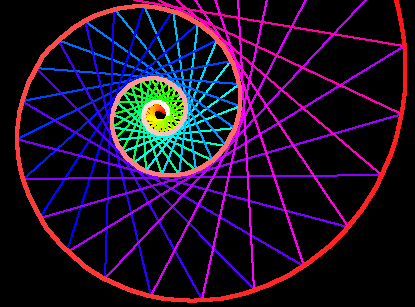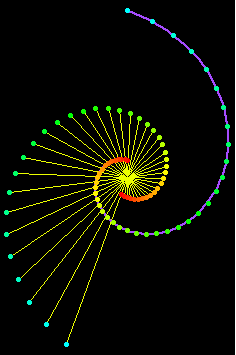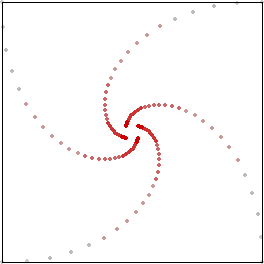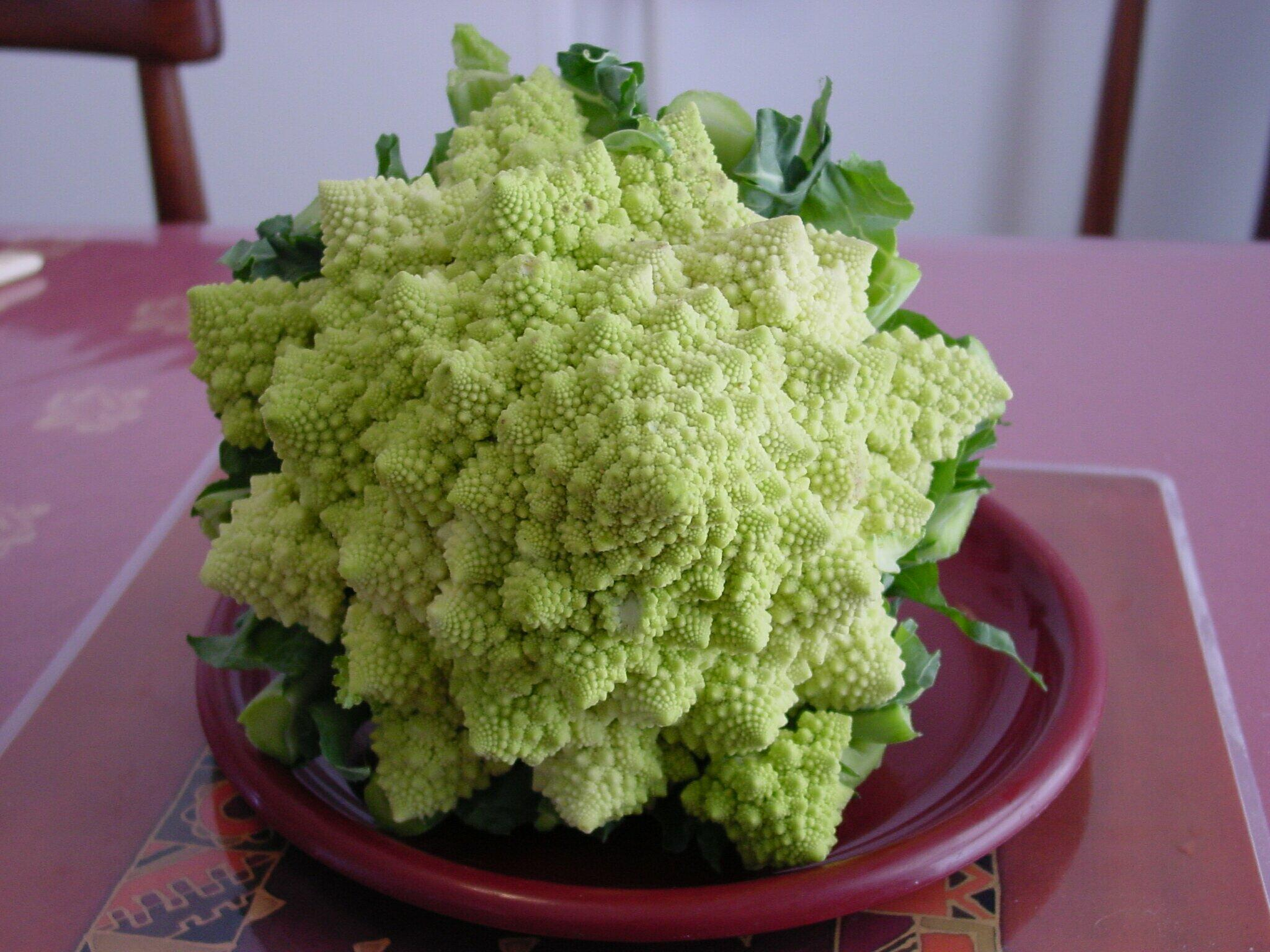# Equiangular SpiralA equiangular spiral and its secants.

## History

The investigation of spirals began at least with the ancient Greeks. The famous Equiangular Spiral was discovered by Rene Descartes, its properties of self-reproduction by Jacob Bernoulli (1654 to 1705) (aka James or Jacques) who requested that the curve be engraved upon his tomb with the phrase “Eadem mutata resurgo” (“I shall arise the same, though changed.”) [Source: Robert C Yates (1952)]

The equiangular spiral was first considered in 1638 by Descartes, who started from the property s = a.r. Evangelista Torricelli, who died in 1647, worked on it independently and used for a definition the fact that the radii are in geometric progression if the angles increase uniformly. From this he discovered the relation s = a.r; that is to say, he found the rectification of the curve. Jacob Bernoulli, some fifty years later, found all the “reproductive” properties of the curve; and these almost mystic properties of the “wonderful” spiral made him wish to have the curve incised on his tomb: Eadem mutata resurgo: “Though changed I rise unchanged”. [source: E H Lockwood (1961)]

## Description

Equiangular spiral describes a family of spirals of one parameter. It is defined as a curve that cuts all radial line at a constant angle.

It also called logarithmic spiral, Bernoulli spiral, and logistique.

Explanation:

1. Let there be a spiral (that is, any curve r==f[θ] where f is a monotonic inscreasing function)
2. From any point P on the spiral, draw a line toward the center of the spiral. (this line is called the radial line)
3. If the angle formed by the radial line and the tangent for any point P is constant, the curve is a equiangular spiral.A example of equiangular spiral with angle 80°.

A special case of equiangular spiral is the circle, where the constant angle is 90°.Equiangular spirals with 40°, 50°, 60°, 70°, 80° and 85°. (left to right) Equiangular Spiral

## Formula

Let α be the constant angle.

Polar: r == E^(θ * Cot[α])

Parametric: E^(t * Cot[α]) {Cos[t],Sin[t]}

Cartesian: x^2 + y^2 == E^(ArcTan[y/x] Cot[α] )

## Properties

### Point Construction and Geometric Sequence

Length of segments of any radial ray cut by the curve is a geometric sequence, with a multiplier of E^(2 π Cot[α]).

Lengths of segments of the curve, cut by equally spaced radial rays, is a geometric sequence.The curve cut by radial rays. The length of any green ray's segments is geometric sequence. The lengths of red segments is also a geometric sequence. In the figure, the dots are points on a 85° equiangular spiral.

### Catacaustic

Catacaustic of a equiangular spiral with light source at center is a equal spiral.

Proof: Let O be the center of the curve. Let α be the curve's constant angle. Let Q be the reflection of O through the tangent normal of a point P on the curve. Consider Triangle[O,P,Q]. For any point P, Length[Segment[O,P]]==Length[Segment[P,Q]] and Angle[O,P,Q] is constant. (Angle[O,P,Q] is constant because the curve's constant angle definition.) Therefore, by argument of similar triangle, then for any point P, Length[Segment[O,Q]]==Length[Segment[O,P]]*s for some constant s. Since scaling and rotation around its center does not change the curve, thus the locus of Q is a equiangular spiral with constant angle α, and Angle[O,Q,P] == α. Line[P,Q] is the tangent at Q.

### Curvature

The evolute of a equiangular spiral is the same spiral rotated.

The involute of a equiangular spiral is the same spiral rotated.Left: Tangent circles of a 80° equiangular spiral. The white dots are the centers of osculating circles, the lines are the radiuses. Right: Lines are the tangent normals, forming the evolute curve by envelope. Equiangular Spiral Evolute

The radial of a equiangular spiral is itself scaled. The figure on the left shows a 70° equiangular spiral and its radial. The figure on the right shows its involute, which is another equiangular spiral.### Inversion

The inversion of a equiangular spiral with respect to its center is a equal spiral.

### Pedal

The pedal of a equiangular spiral with respect to its center is a equal spiral.Pedal of a equiangular spiral. The lines from center to the red dots is perpendicular to the tangents (blue lines). The blue curve is a 60° equiangular spiral. The red dots forms its pedal.

### Pursuit Curve

Persuit curves are the trace of a object chasing another. Suppose there are n bugs each at a corner of a n sided regular polygon. Each bug crawls towards its next neighbor with uniform speed. The trace of these bugs are equiangular spirals of (n-2)/n * π/2 radians (half the angle of the polygon's corner).Left: shows the trace of four bugs, resulting four equiangular spirals of 45°. Above right: six objects forming a chasing chain. Each line is the direction of movement and is tangent to the equiangular spirals so formed.

### Spiral in nature

Spiral is the basis for many natural growths. Seashells have the geometry of equiangular spiral.A cauliflower (Romanesco broccoli) exhibiting equiangular spiral and fractal geometry. (Photo by Dror Bar-Natan. http://www.math.toronto.edu/%7Edrorbn/Gallery/Plants/index.html)

## Related Web Sites

Robert Yates: Curves and Their Properties .

Khristo Boyadzhiev, Spirals and Conchospirals in the Flight of Insects. The College Mathematics Journal, Jan 1999. Khristo_Boyadzhiev_CMJ-99.pdf

The MacTutor History of Mathematics archive